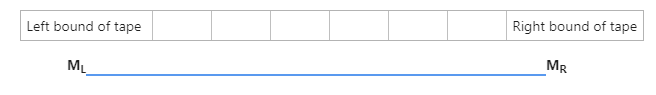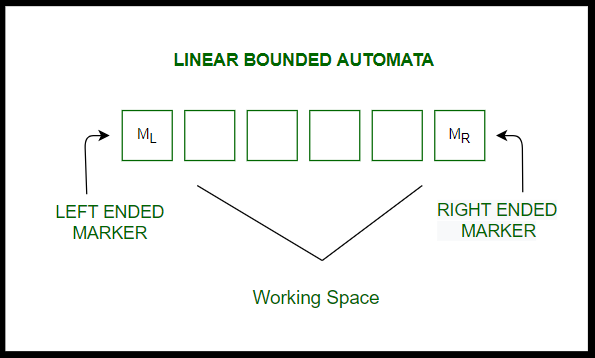Related Articles
Introduction to Linear Bounded Automata (LBA)
• Last Updated : 02 Mar, 2021

History :
In 1960, associate degree automaton model was introduced by Myhill and these days this automation model is understood as deterministic linear bounded automaton. After this, another scientist named Landweber worked on this and proposed that the languages accepted by a deterministic LBA are continually context-sensitive languages.

In 1964, Kuroda introduced a replacement and a lot of general models specially for non-deterministic linear bounded automata, and established that the languages accepted by the non-deterministic linear bounded automata are exactly the context-sensitive languages.

Introduction to Linear Bounded Automata :
A Linear Bounded Automaton (LBA) is similar to Turing Machine with some properties stated below:

• Turing Machine with Non-deterministic logic,
• Turing Machine with Multi-track, and
• Turing Machine with a bounded finite length of the tape.Tuples Used in LBA :
LBA can be defined with eight tuples (elements that help to design automata) as:

```M = (Q , T , E , q0 , ML , MR , S , F),

where,
Q -> A finite set of transition states
T -> Tape alphabet
E -> Input alphabet
q0 -> Initial state
ML -> Left bound of tape
MR -> Right bound of tape
S -> Transition Function
F -> A finite set of final states ```

Diagrammatic Representation of LBA :Examples:

Languages that form LBA with tape as shown above,

• L = {an! | n >= 0}
• L = {wn | w from {a, b}+, n >= 1}
• L = {wwwR | w from {a, b}+}

Facts :

```Suppose that a given LBA M has
--> q states,
--> m characters within the tape alphabet, and
--> the input length is n```
1. Then M can be in at most f(n) = q * n * mn configurations i.e. a tape of n cells and m symbols, we are able to have solely mn totally different tapes.
2. The tape head is typically on any of the n cells which we have a tendency to are typically death penalty in any of the q states.
My Personal Notes arrow_drop_up